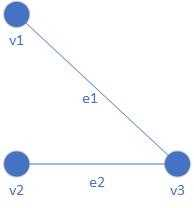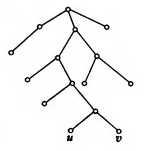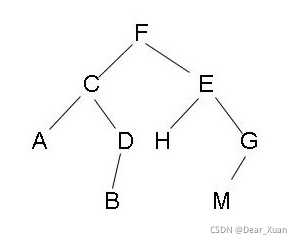## 图的基本概念

### 无向图

V是非空有穷集,称为顶点集,里面的元素称为顶点

E是可以为空的有穷集,称为边集,里面的元素称为无向边(简称 边)$e_1=\{v_1,v_3\},e_2=\{v_2,v_3\}$

$V=\{v_1,v_2,v_3\},E=\{e_1,e_2\}$

$$即为无向图$G$

### 图的属性

$V(G)$$E(G)$分别表示G的顶点集和边集,$|V(G)|$$|E(G)|$分别表示$G$的顶点数和边数

### 通路与回路

G中顶点与边的交替序列Γ称为通路

$d$是从u到v的最短通路

## 树

### 无向树

$G=$是n阶m条边的无向图,那么下面的命题都是等价的,也就是说只要知道其中一个就能推出别的所有命题

1. G是树
2. G中任意两个顶点存在唯一路径
3. G中无回路且$m=n-1$
4. G中是连通的且$m=n-1$
5. G中没有回路,但是在任意两个不同的顶点之间加一条边后所得的图中有唯一的一个含新边的圈

### 根树## 二叉树

### 二叉树的概念